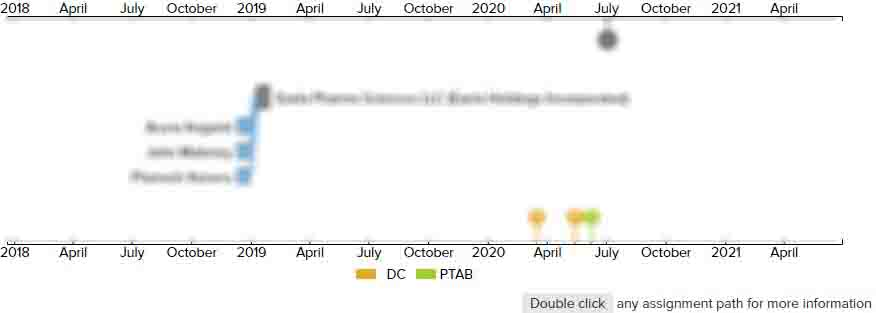# SYSTEMS AND METHODS FOR DETERMINING INTRAOCULAR LENS POWER

• US 20090251664A1
• Filed: 04/06/2009
• Published: 10/08/2009
• Est. Priority Date: 04/04/2008
• Status: Active Grant
##### First Claim
Patent Images

1. A method of selecting an intraocular lens or an optical power thereof, comprising:

• providing an eye comprising a cornea and a retina;

determining a value of an axial length of an eye, the axial length being equal to a distance from the apex to a surface of the retina;

calculating, based on a mathematical relationship, a distance from the apex to a plane the intraocular lens after an ocular surgical procedure;

calculating an optical power of the intraocular lens suitable for providing a predetermined refractive outcome;

wherein the mathematical relationship comprises a dependent variable that varies with a value of an independent variable, the independent variable having a base value corresponding to the determined axial length;

wherein the mathematical relationship has a sensitivity of less than or equal to 0.5%, the sensitivity being defined as a percent variation in the dependent variable for a 1% in the base value.

• ##### 3 Assignments
Timeline View
Assignment View×
×